# C# 表达式树 创建、生成、使用、lambda转成表达式树~表达式树的知识详解### 表达式树的概念

• 表达式树的创建有 Lambda法组装法
• 学习表达式树需要 委托、Lambda、Func<> 基础。
• 表达式树 形状可以参考 二叉树。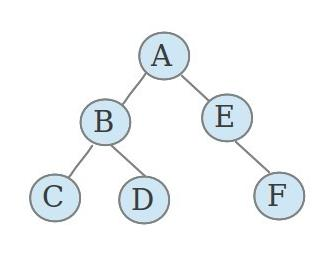• 可以把表达式树理解成 数学表达式。

数学表达式的所有常量、符号为表达式树的底节点。每一次计算生成的结果是一个结点，或者说他们的共同结点就是他们应该进行的运算。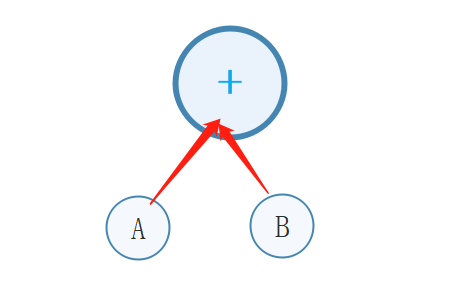## 生成表达式树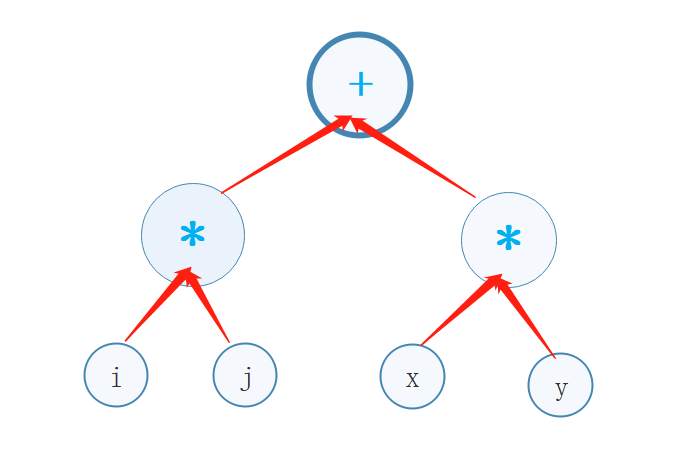### Lambda 生成表达式树

在控制台创建应用，需要引入

```using System.Linq.Expressions;
```

1，创建表达式

(系统自动把 Lambda表达式 转为表达式树，当然，不是所有的 Lambda表达式都能转为表达式树，详细请参考文章后面的“系统自动把 Lambda表达式 转为 表达式树” 一节)

``` Expression<Func<int, int, int, int, int>> func = (i, j, x, y) => (i * j) + (x * y);
```

2，输出系统转换的表达式

输入这一行代码后运行，看看控制台输出的表达式树

```Console.WriteLine(func);
```

3，把代码转为数据

(把代码当作数据来使用)

```var compile = func.Compile();
//或 Func<int, int, int, int, int> compile = func.Compile();
```

4，代入运算

```            int result = compile(12, 13, 14, 15);       //把具体数字代入表达式并运算
Console.WriteLine(result);      //输出表达式结果```

```           Expression<Func<int, int, int, int, int>> func = (i, j, x, y) => (i * j) + (x * y);
Console.WriteLine(func);        //输出表达式

var compile = func.Compile();       //把代码转为数据
//或 Func<int, int, int, int, int> compile = func.Compile();

int result = compile(12, 13, 14, 15);       //把具体数字代入表达式并运算
Console.WriteLine(result);      //输出表达式结果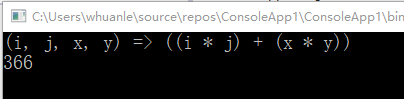### 组装法生成表达式树

1，生成 a b  d 参数

```　　　　　　  ParameterExpression a = Expression.Parameter(typeof(int), "i");
ParameterExpression b = Expression.Parameter(typeof(int), "j");
ParameterExpression c = Expression.Parameter(typeof(int), "x");
ParameterExpression d = Expression.Parameter(typeof(int), "y");```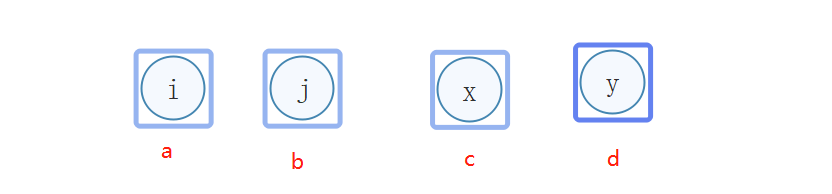分析：

i、j、x、y 是结点名称，a、b、c、d 是实例名称。不用留精力思考我上面 a b c d i j x y 的名称设定。

ParameterExpression 表示创建一个节点，Parameter 表示一个命名的参数表达式，详细请参考文章后面的 “Expression 参数分类”

Expression.Parameter(Type type,string name) 表示这个节点的属性。

2，生成结点

```            Expression r1 = Expression.Multiply(a, b);      //乘法运行
Expression r2 = Expression.Multiply(c, d);      //乘法运行```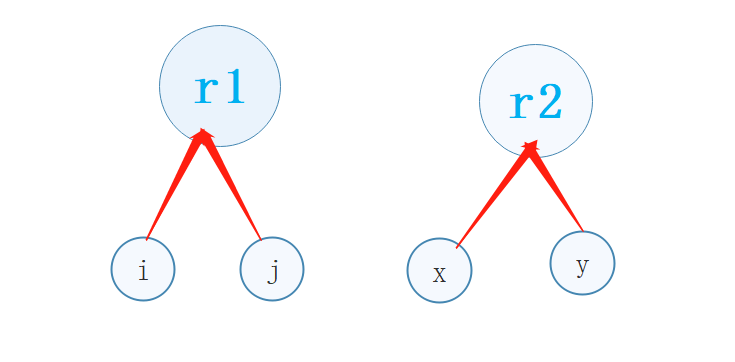分析：

创建了 ( i * j )( x * y ) 两个运算

Multiply 表示 不进行溢出检查的乘法运算。Expression 里有 85种 操作方法，更多加减乘除比较大小等操作在文章后面详细附上，参考 “ 运算操作符” 一节。

3，生成终结点

```Expression result = Expression.Add(r1, r2);     //相加
```

4，生成表达式树、转换、输出表达式树、代入数据进行运算

```            Expression<Func<int, int, int, int, int>> func = Expression.Lambda<Func<int, int, int, int, int>>(result, a, b, c, d);
var com = func.Compile();
Console.WriteLine("表达式" + func);
Console.WriteLine(com(12, 12, 13, 13));```

```            ParameterExpression a = Expression.Parameter(typeof(int), "i");
ParameterExpression b = Expression.Parameter(typeof(int), "j");

Expression r1 = Expression.Multiply(a, b);      //乘法运行
ParameterExpression c = Expression.Parameter(typeof(int), "x");
ParameterExpression d = Expression.Parameter(typeof(int), "y");
Expression r2 = Expression.Multiply(c, d);      //乘法运行

Expression result = Expression.Add(r1, r2);     //相加
//以上代码产生结点
//生成表达式
Expression<Func<int, int, int, int, int>> func = Expression.Lambda<Func<int, int, int, int, int>>(result, a, b, c, d);
var com = func.Compile();
Console.WriteLine("表达式" + func);
Console.WriteLine(com(12, 12, 13, 13));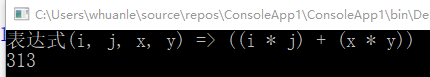## 补充说明

### 1，系统自动把 Lambda表达式 转为 表达式树

对 lambda表达式 的要求 只能 由 传入参数 和 返回参数 两部分表示。lambda表达式 不能包含其它判断、循环等的代码。

错误举例```            Expression<Func<int, int, int, int, int>> func = (a, b, c, d) =>
{
if (a < 10)
{
a += 1;
}
/*
* 其它操作代码
*/
return a + b + c + d;
};```

View Code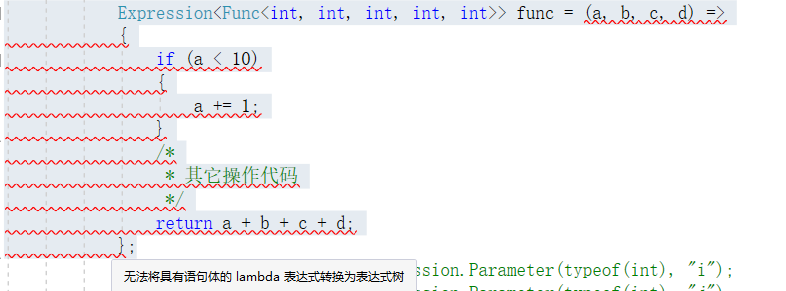把那些东西通通删除，修改后：

``` Expression<Func<int, int, int, int, int>> func = (a, b, c, d) => a + b + c + d;
```

这样的 “最简” 的 lambda表达式 才能被系统自动转为表达式树

### 2，运算操作符

一般数学上，有加减乘除、取余、求幂等操作，而在程序中，运算操作符可以有更多的选择，达 85 种。

笔者这里给出一张图列出部分方法。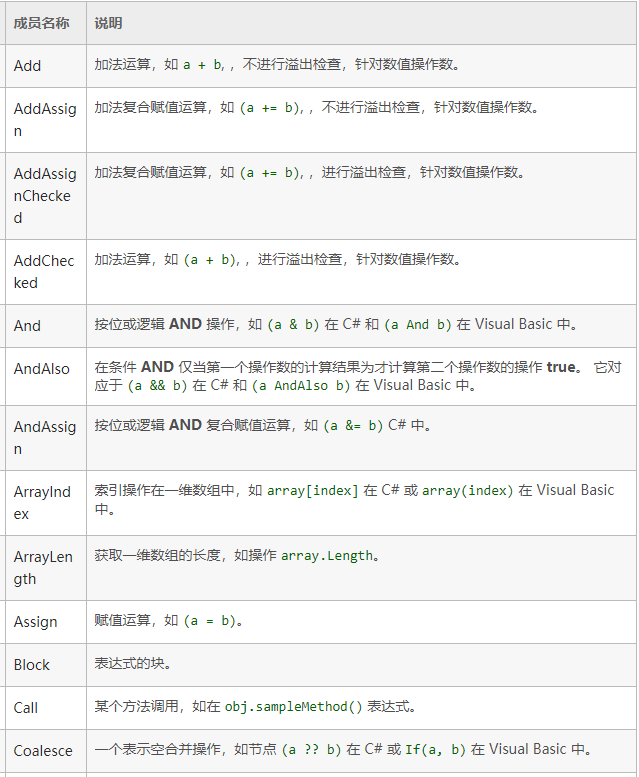### 3，Expression 参数

Parameter 类似于 数学的 未知数 如 a 、 b；使用方法

`ParameterExpression a = Expression.Parameter(typeof(int), "a")`
`ParameterExpression b = Expression.Parameter(typeof(int), "b")`

Constant 表示一个常数，例如 2πb 中的 2 或者 2π ；使用方法

```ConstantExpression define = Expression.Constant(2);
```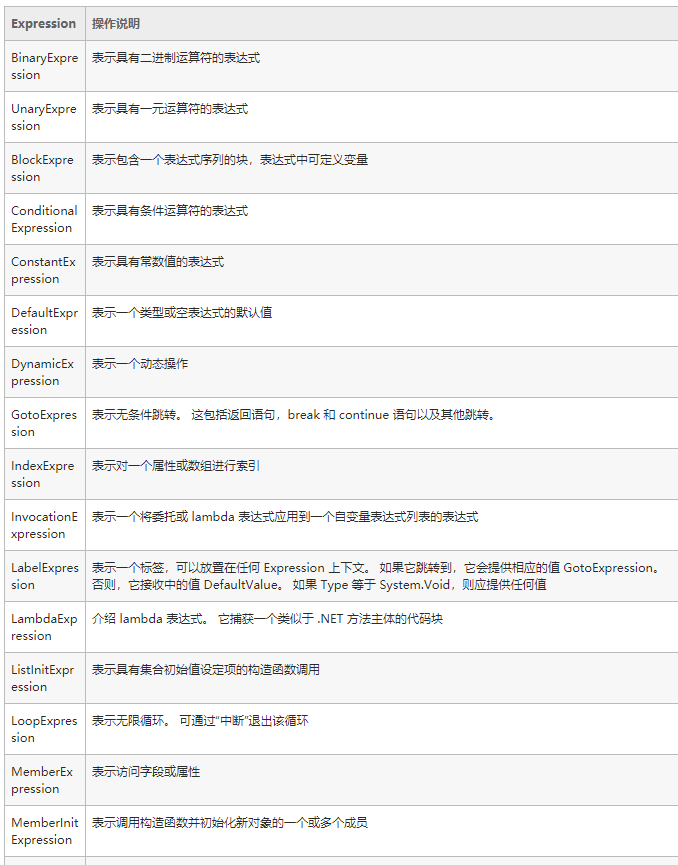### 4，Expression 的操作方法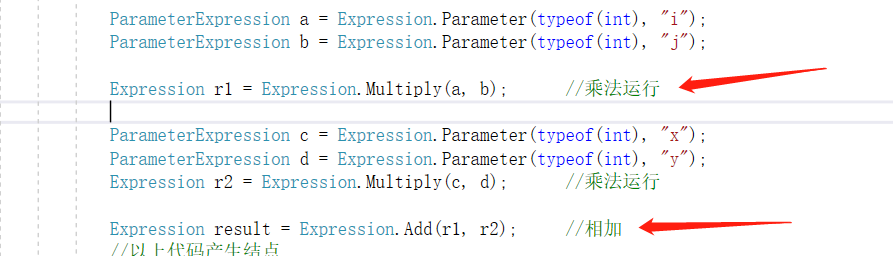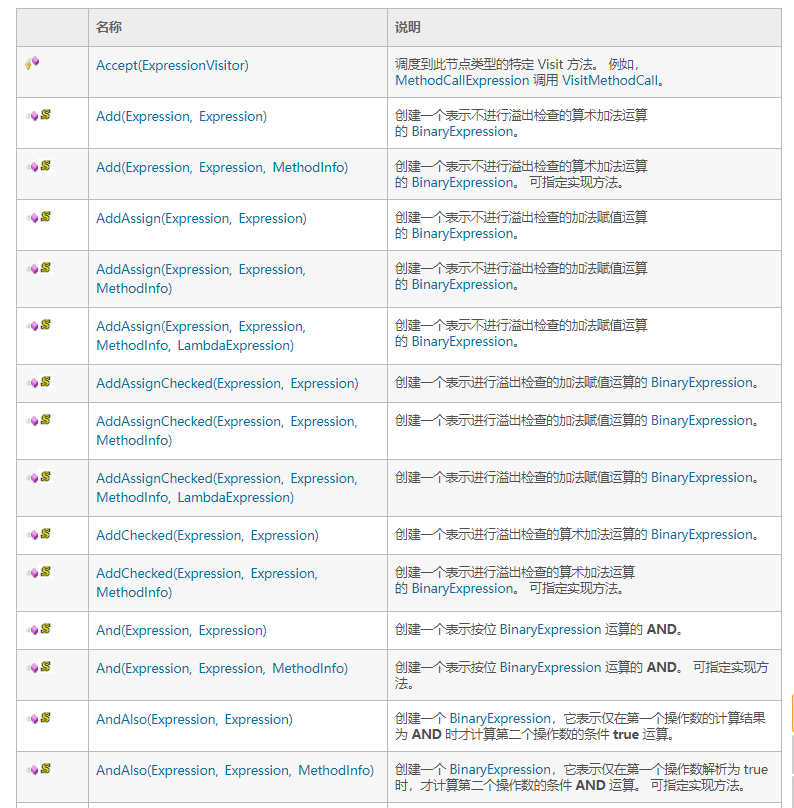### 5，表达式树的高级用法

1.lexapro online说道：

I'm just commenting to let you be aware of of the fabulous discovery my cousin's daughter experienced going through your site. She even learned lots of issues, most notably how it is like to have a very effective giving mindset to have many others just gain knowledge of chosen grueling matters. You truly surpassed people's expected results. Thank you for producing these precious, trusted, informative and in addition fun tips about this topic to Lizeth.